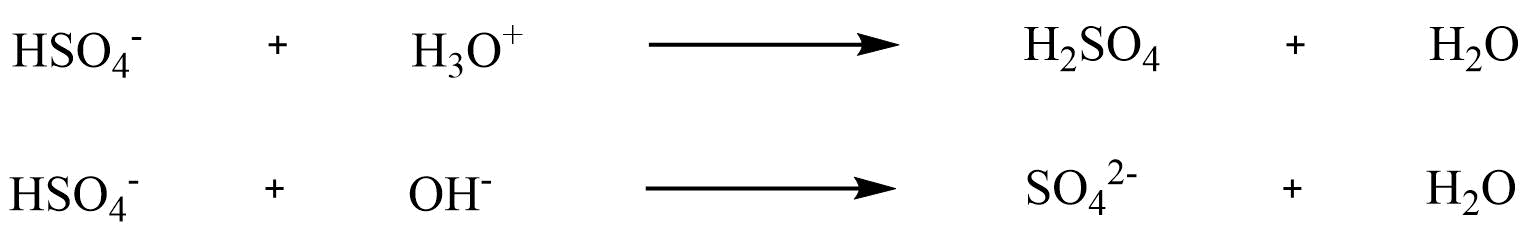## Acid and Base Strength

### Objectives

After completing this section, you should be able to

1. state the Brønsted-Lowry definition of an acid and a base.
2. identify the Brønsted-Lowry acid and base in a given acid-base reaction.

### Key Terms

Make certain that you can define, and use in context, the key terms below.

• acid (Brønsted-Lowry)
• base (Brønsted-Lowry)
• conjugate acid
• conjugate base

### Study Notes

You should be familiar with the Brønsted-Lowry concept of acidity and the differences between strong and weak acids. You may wish to review this topic before proceeding.

In 1923, chemists Johannes Brønsted and Martin Lowry independently developed definitions of acids and bases based on compounds abilities to either donate or accept protons (H+ ions). Here, acids are defined as being able to donate protons in the form of hydrogen ions; whereas bases are defined as being able to accept protons. This took the Arrhenius definition one step further as water is no longer required to be present in the solution for acid and base reactions to occur.

### Brønsted-Lowery Definition

J.N. Brønsted and T.M. Lowry independently developed the theory of proton donors and proton acceptors in acid-base reactions, coincidentally in the same region and during the same year. The Arrhenius theory where acids and bases are defined by whether the molecule contains hydrogen and hydroxide ion is too limiting. The main effect of the Brønsted-Lowry definition is to identify the proton (H+) transfer occurring in the acid-base reaction. This is best illustrated in the following equation:

HA+Z⇌A−+HZ+
 Acid Base Donates hydrogen ions Accepts hydrogen ions. HCl+ HOH → H3O+ + Cl– HOH+ NH3→ NH4+ + OH–

The determination of a substance as a Brønsted-Lowery acid or base can only be done by observing the reaction. In the case of the HOH it is a base in the first case and an acid in the second case.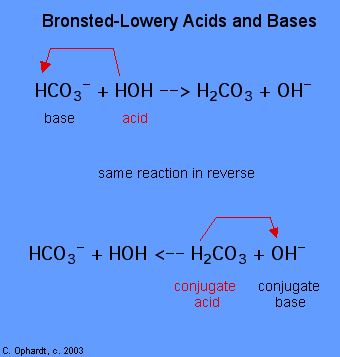To determine whether a substance is an acid or a base, count the hydrogens on each substance before and after the reaction. If the number of hydrogens has decreased that substance is the acid (donates hydrogen ions). If the number of hydrogens has increased that substance is the base (accepts hydrogen ions). These definitions are normally applied to the reactants on the left. If the reaction is viewed in reverse a new acid and base can be identified. The substances on the right side of the equation are called conjugate acid and conjugate base compared to those on the left. Also note that the original acid turns in the conjugate base after the reaction is over.

### Acids are Proton Donors and Bases are Proton Acceptors

For a reaction to be in equilibrium a transfer of electrons needs to occur. The acid will give an electron away and the base will receive the electron. Acids and Bases that work together in this fashion are called a conjugate pair made up of conjugate acids and conjugate bases.HA+Z⇌A−+HZ+

A stands for an Acidic compound and Z stands for a Basic compound

• A Donates H to form HZ+.
• Z Accepts H from A which forms HZ+
• A becomes conjugate base of HA and in the reverse reaction it accepts a H from HZ to recreate HA in order to remain in equilibrium
• HZ+ becomes a conjugate acid of Z and in the reverse reaction it donates a H to A recreating Z in order to remain in equilibrium

### Questions

1. Why is HA an Acid?
2. Why is A a Base?
3. How can A be a base when HA was and Acid?
4. How can HZ+ be an acid when Z used to be a Base?
5. Now that we understand the concept, let’s look at an an example with actual compounds!
HCl+H2O⇌H3O++Cl¯
• HCL is the acid because it is donating a proton to H2O
• H2O is the base because H2O is accepting a proton from HCL
• H3O+ is the conjugate acid because it is donating an acid to CL turn into it’s conjugate acid H2O
• Cl¯ is the conjugate base because it accepts an H from H3O to return to it’s conjugate acid HCl

How can H2O be a base? I thought it was neutral?

### Conjugate Acid–Base Pairs

We discussed the concept of conjugate acid–base pairs in Chapter 4, using the reaction of ammonia, the base, with water, the acid, as an example. In aqueous solutions, acids and bases can be defined in terms of the transfer of a proton from an acid to a base. Thus for every acidic species in an aqueous solution, there exists a species derived from the acid by the loss of a proton. These two species that differ by only a proton constitute a conjugate acid–base pair. For example, in the reaction of HCl with water (Equation 16.1), HCl , the parent acid, donates a proton to a water molecule, the parent base, thereby forming Cl. Thus Cl and Cl constitute a conjugate acid–base pair. By convention, we always write a conjugate acid–base pair as the acid followed by its conjugate base. In the reverse reaction, the Cl ion in solution acts as a base to accept a proton from H3O+, forming H2O and HCl. Thus H3O+ and H2O constitute a second conjugate acid–base pair. In general, any acid–base reaction must contain two conjugate acid–base pairs, which in this case are HCl/Cl and H3O+/H2O.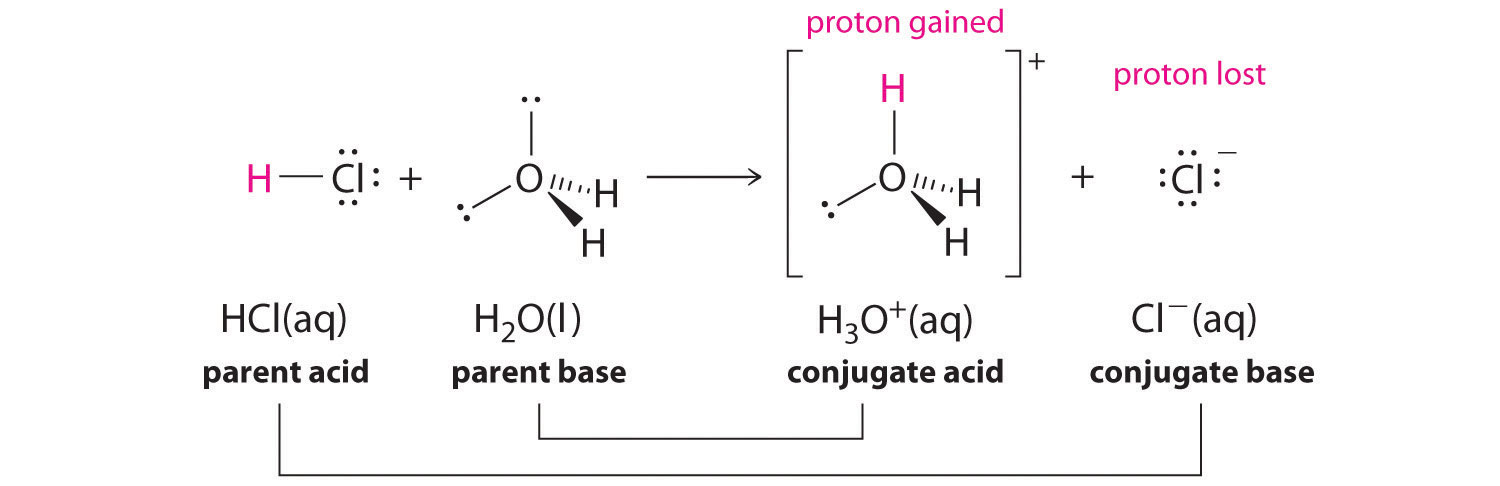Note

All acid–base reactions contain two conjugate acid–base pairs.

Similarly, in the reaction of acetic acid with water, acetic acid donates a proton to water, which acts as the base. In the reverse reaction, H3O+ is the acid that donates a proton to the acetate ion, which acts as the base. Once again, we have two conjugate acid–base pairs: the parent acid and its conjugate base (CH3CO2H/CH3CO2) and the parent base and its conjugate acid (H3O+/H2O).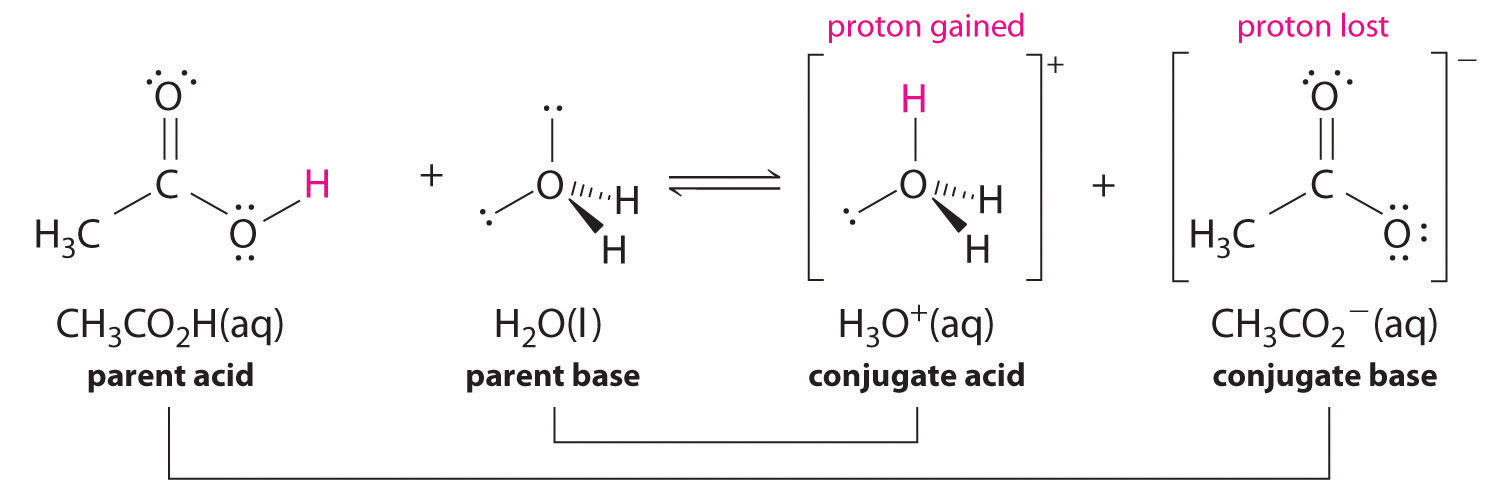In the reaction of ammonia with water to give ammonium ions and hydroxide ions (Equation 16.3), ammonia acts as a base by accepting a proton from a water molecule, which in this case means that water is acting as an acid. In the reverse reaction, an ammonium ion acts as an acid by donating a proton to a hydroxide ion, and the hydroxide ion acts as a base. The conjugate acid–base pairs for this reaction are NH4+/NH3 and H2O/OH. Some common conjugate acid–base pairs are shown in Figure 2.7.1.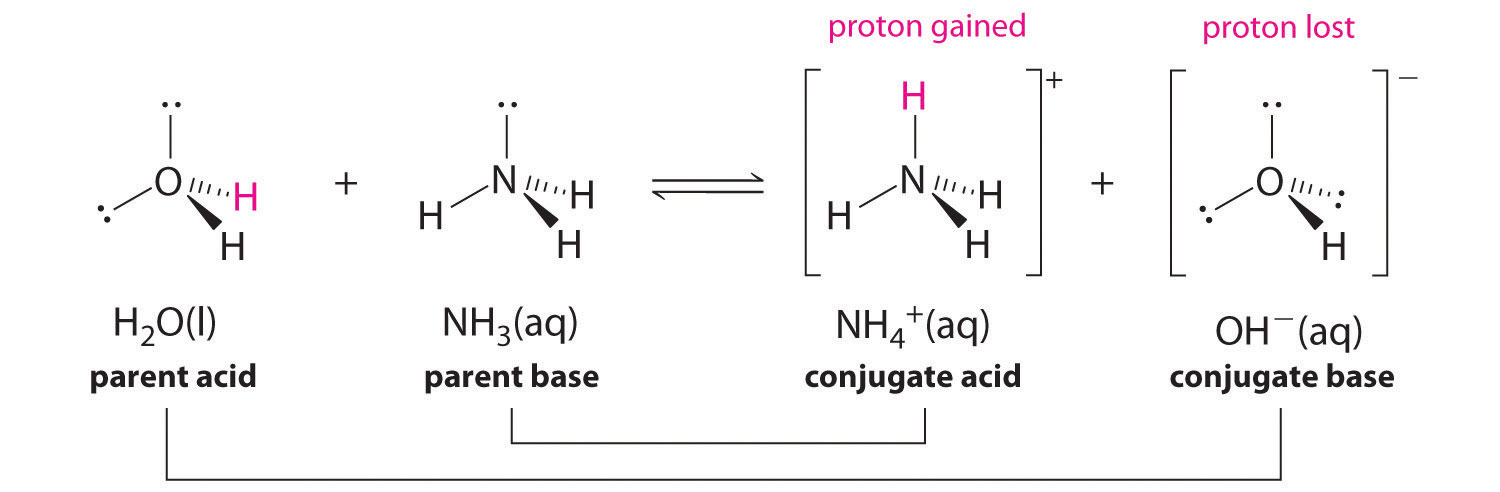Figure 1: The Relative Strengths of Some Common Conjugate Acid–Base Pairs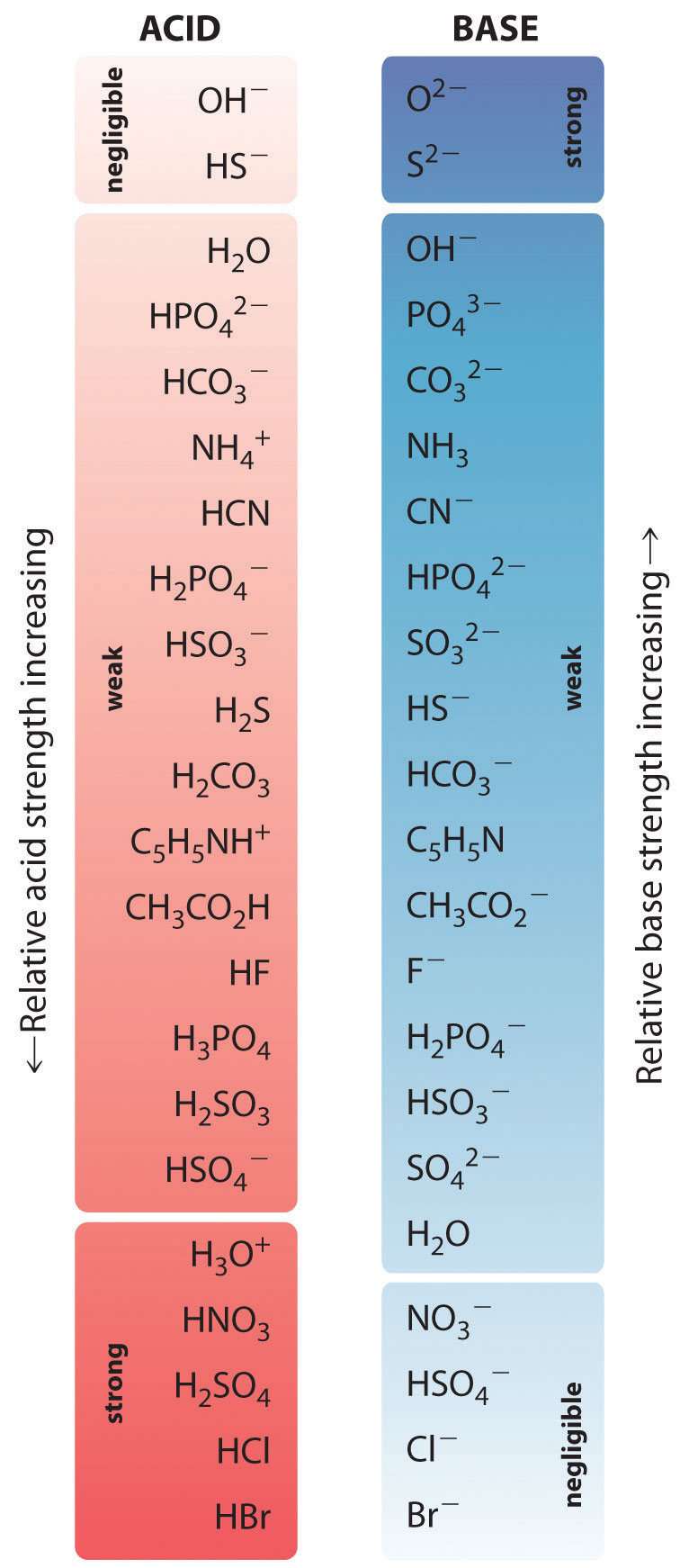The strongest acids are at the bottom left, and the strongest bases are at the top right. The conjugate base of a strong acid is a very weak base, and, conversely, the conjugate acid of a strong base is a very weak acid.

### Exercises

1. Identify the Brønsted-Lowry acids and bases in the reactions given below.
1. $\ce{\sf{CH3CH2O^- + H2O <=> CH3CH2OH + OH^- }}$
2. $\ce{\sf{CH3CH2OH + H2SO4 <=> CH3CH2OH2+ + HSO4- }}$

2. Is the following molecule a Brønsted acid or base?

HSO4

#### Solutions

1.2.2.

It can be both, consider the following schemes: